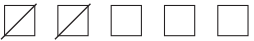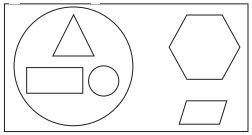Students can download 5th Maths Term 3 Chapter 6 Fractions InText Questions and Answers, Notes, Samacheer Kalvi 5th Maths Guide Pdf helps you to revise the complete Tamilnadu State Board New Syllabus, helps students complete homework assignments and to score high marks in board exams.

## Tamilnadu Samacheer Kalvi 5th Maths Solutions Term 3 Chapter 6 Fractions InText Questions

Try This (Text Book Page No. 47)

Question 1.
Represent the crossed out part as a fraction.$$\frac { 2 }{ 5 }$$Question 2.
Represent the figures that are outside the circle as a fraction.$$\frac { 2 }{ 5 }$$
(i) In $$\frac { 3 }{ 7 }$$, ____ is the numerator and ___ is the denominator.(ii) In $$\frac { 6 }{ 10 }$$, ____ is the numerator and ___ is the denominator.